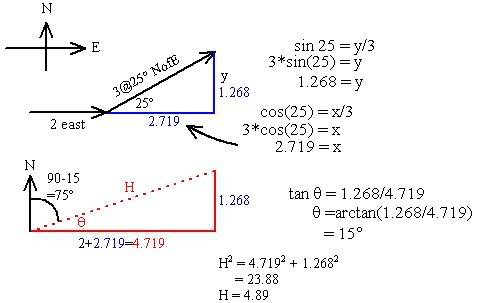# What is the net force on the object?

1irishman

## Homework Statement

A force of 2N east and a force of 3N 25degrees N of E act on an object. What is the net force on the object?
The answer according to the text is 4.9N 75Degrees E of N

## The Attempt at a Solution

First express direction in terms of x-axis:

Resolve 2N East into x and y components

Rx = R cos Theta Ry = R sin Theta
=2Ncos180 =2Nsin180
= -1.9N =0

Resolve 3N 25degrees N of E into x and y components

Rx = R cos Theta Ry = R sin Theta
=3cos335degrees =3sin335degrees
=1.6N = -2.6N

Rx=R1x+R2x
= -1.9N + 1.6N
= -0.3N west direction because of minus sign

Ry=R1y+R2y
=0N + -2.6N
= -2.6N south direction because of minus sign

Add Rx and Ry to obtain R

R= sqrt Rx^2 + Ry^2
= -0.3^2 + -2.6^2
= sqrt of 6.85
= 2.62N

arc tan -0.3/-2.6= theta

## Answers and Replies

Homework Helper
I get the 4.89 N answer.
When you resolve 3@25 degrees NofE, you get
(3*cos25,3*sin25) = (2.719, 1.268)
When you add the (2,0) to that you get (4.719,1.268).

1irishman
When i resolve 3@25 degrees NofE, I get
(3*cos25, 3*sin25) = (2.772, 1.148)
Then for 2N I added what I got (to the above) which was (1.85, 0.76) to get (4.622, 1.908)
I got 4.9N, but I don't know how they got 75 degrees east of north?

Staff Emeritus
Gold Member

## The Attempt at a Solution

First express direction in terms of x-axis:

Resolve 2N East into x and y components

Rx = R cos Theta Ry = R sin Theta
=2Ncos180 =2Nsin180
= -1.9N =0

Well this is not making any sense at all for numerous reasons.

Reason 1: Normally you'd define your coordinate system so that east was the positive x-direction and north was the positive y-direction. If so, then there is no need to resolve this vector into components, because you already know that it points entirely in the x-direction (east), therefore its components are obviously (2,0) by inspection.

Reason 2: Even if you were going to go through the wasted effort to calculate the components explicitly, the angle is measured from the positive x-axis, meaning that it should be 0 degrees, not 180 degrees.

Reason 3: Even if you were to use 180 degrees for some strange reason, the cosine of 180 degrees is -1. Can you explain to me how 2*-1 could possibly be equal to -1.9 ???

Reason 4: Your formatting (spacing) didn't work out properly in the final post, so that it looks like you are writing 2N*cos180 = 2N*sin180 and 1.9 N = 0 N, both of which are nonsensical. I just thought I'd point that out, as I was quite confused by your equalities before I realised that there was formatting issue.

Homework Helper
Sketch (4.622, 1.908) and its hypotenuse. Use trig to find the angle. You should get 15 degrees. But this is north of east. So you have 90-15 degrees east of north.

1irishman
I drew the triangle with base 4.622units and height 1.908units and got the hypotenuse is 5units then i got arc tan 1.908/4.622 which was 24degrees? I'm so confused now! Help?

Staff Emeritus
Gold Member
I drew the triangle with base 4.622units and height 1.908units and got the hypotenuse is 5units then i got arc tan 1.908/4.622 which was 24degrees? I'm so confused now! Help?

First of all, (4.622,1.908) isn't even the right answer, because of the issues that I identified. Second, whatever angle you get will be north of east (clockwise from the positive x-axis). The angle measured east of north will be its complement.

1irishman
It has been 19 years since I have looked at Physics or Trigonometry. I come to these forums, show the best solution I have come up with based on my very limited experience, and often get useful ideas, hints etc that help me come up with the solution myself. I appreciate the depth of your obvious knowledge in this subject area, but sometimes newbies such as myself need baby steps initially until we get used to thinking about the concepts herein. Comments like "for some strange reason" don't usually offer anything valuable to the learning process in my opinion.

Homework Helper
Sorry about that, irishman. We have to be careful about helping too much and sometimes fail to help enough. Here is the all-important diagram and complete solution.The upper diagram shows the two vectors placed head-to-tail; the 3 added onto the end of the 2. The total is the new vector going from beginning to end of that chain, but it is awkward to show on the same diagram so I've made a second diagram to show that total vector. From the calcs in the first diagram, we know the x and y components. I used the tangent to find the angle and the pythagorean formula to find the length.